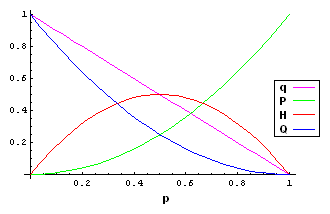# EvoMath 2: Testing for Hardy-Weinberg Equilibrium

In the first installment of EvoMath, I derived the Hardy-Weinberg Principle and discussed its significance to biology. In the second installment I will demonstrate how to test if a population deviates from Hardy-Weinberg equilibrium.

## Recap:

A population is considered to be in Hardy-Weinberg equilibrium if the allele and genotype frequencies are as follows.

 Genotype Frequency AA $$P = P^\prime = p^2$$ Aa $$H = H^\prime = 2pq$$ aa $$Q = Q^\prime = q^2$$
 Allele Frequency A $$p = P + \frac{H}{2}$$ a $$q = 1-p = Q + \frac{H}{2}$$## Test Procedure:

A goodness-of-fit test can be used to determine if a population is significantly different from the expections of Hardy-Weinberg equilibrium. If we have a series of genotype counts from a population, then we can compare these counts to the ones predicted by the Hardy-Weinberg model. We conclude that the population is not in Hardy-Weinberg equilibrium if the probability that the counts were drawn under the Hardy-Weinberg model is too small for the deviations to be considered due to random chance. The significance level that is typically used is $$\alpha = 0.05$$, i.e. the genotype counts have less than a one in twenty chance of being caused by a population in Hardy-Weinberg equilibrium.

In order to calculate this probability, we will use a test statistic, $$\chi^2$$, which was devised in 1900 by Karl Pearson and has a well characterized distribution. If $$O_i$$ are the set of observed counts, and $$E_i$$ are the set of expected counts, then

$\chi^2 = \sum_i{\frac{ \left( O_i-E_i \right)^2 }{ E_i }}.$

This test statistic has a “chi-square” distribution with $$\nu$$ degrees of freedom. Since we are testing Hardy-Weinberg equilibrium with two alleles, $$\nu=1$$ (rationale not shown). Furthermore, it can be shown that if $$\chi^2 \ge 3.841$$ then $$\Pr{ \left\{ \chi^2 \right\} } \le 0.05$$. Therefore, if $$\chi^2 \ge 3.841$$ we will reject the null model and conclude that there is significant statistical support that the population is not in Hardy-Weinberg equilibrium.

## Example 1:

Consider the following samples from a population.

 Genotype Count AA 30 Aa 55 aa 15
 Allele Frequency A 0.575 a 0.425

Calculate the $$\chi^2$$ value.

 Genotype Observed Expected (O-E)2/E AA 30 33 0.27 Aa 55 49 0.73 aa 25 18 0.50 Total 100 100 1.50

Since $$\chi^2 = 1.50 < 3.841$$, we conclude that the genotype frequencies in this population are not significantly different than what would be expected if the population is in Hardy-Weinberg equilibrium.

## Example 2:

Race and Sanger (1975) determined the blood groups of 1000 Britons as follows (from Hartl and Clarke 1997).

 Genotype Observed Expected MM 298 294.3 MN 489 496.4 NN 213 209.3

This results in $$\chi^2 = 0.222 < 3.841$$. As in the previous example, the measured genotype frequencies are not significantly different from the expectations of Hardy-Weinberg equilibrium.

## Example 3:

Matthijis et al. (1998) surveyed a group of 54 people suffering from Jaeken syndrome (from Freeman and Herron 2004).

 Genotype Observed Expected OO 11 19.44 OR 43 25.92 RR 0 8.64

This results in $$\chi^2 = 23.56 > 3.841$$. Unlike the previous two examples, the measured genotype frequencies are significantly different from the expectations of Hardy-Weinberg equilibrium. This indicates that one or more of the Hardy-Weinberg conditions are being violated; although, it does not tell us which ones.

## Conclusion:

Although to derive the Hardy-Weinberg principle, we assumed that the size of the population was infinite, these statistical tests demonstrate that finite populations can approximately exist in Hardy-Weinberg equilibrium.

• Freeman S and Herron JC (2004) Evolutionary Analysis 3rd ed. Pearson Education, Inc (Upper Saddle River, NJ)
• Hartl DL and Clarke AG (1997) Principles of Population Genetics 3rd ed. Sinauer Associates, Inc (Sutherland, MA)
• Matthijis GE et al. (1998) Lack of homozygotes for the most frequent disease allele in carbohydrate-deficient-glycoprotein syndrome type 1A. American Journal of Human Genetics 62: 542-550
• Race RR and Sanger R (1975) Blood Groups in Man 6th ed. JB Lippincott, Philadelphia